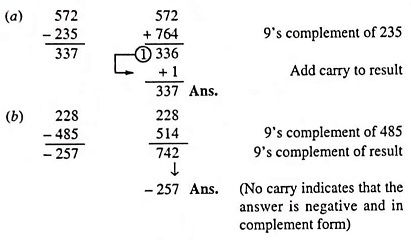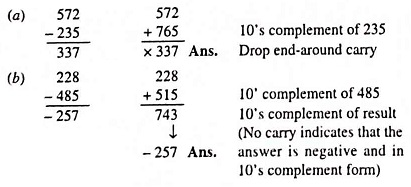## Decimal Number System (Definition, Conversions & Examples):

The decimal number system, as already mentioned, has a base of 10 and is a position-value system. The statement ‘the decimal number system has a base of 10’ implies that it contains ten unique symbols (or digits) i.e., 0, 1, 2, 3, 4, 5, 6, 7, 8 and 9. The ten digits do not limit us to express only ten different quantities because we use the various digits in appropriate positions within a number to indicate the magnitude of the quantity. For expressing quantities exceeding nine, two or more digits are used, and the position of each digit within the number indicates the magnitude it represents. The placement of the digits in the sequenced order from right to left is understood to carry a specific meaning. For instance consider the number 6,523 (six thousand five hundred and twenty three). The total value of this number is obtained by adding 3 unit values, 2 tens, 5 hundreds, and 6 thousands. Expressed more formally, it can be written as

6523 = 6 x 103 + 5 x 102 + 2 x 101 + 3 x 100

The figure 10 plays the role of a base or radix, hence the name decimal number system. The powers of 10 are incremented from zero by one as the position is moved successively toward the left from the decimal point. Furthermore, the coefficients of the powers of 10 are allowed to take on values from 0 to 9.

It will be noted that in number 6523, 3 is the least significant digit (LSD) whereas 6 is the most significant digit (MSD).

### 9’s Complements:

The 9’s complement of a decimal number is determined by subtracting each digit in the number from nine. For example the 9’s complements of 7, 25, 321, 2435 and 57364 are 9-7 i.e., 2, 99-25 i.e., 74, 999-321 i.e., 678, 9999-2435 i.e., 7564 and 99999-57364 i.e., 42635 respectively.

### 9’s Complement Subtraction:

The usefulness of the 9’s complement stems from the fact that subtraction of a smaller decimal number from a larger one can be accomplished by adding the 9 ‘s complement of the subtrahend (in this case the smaller number) to the minuend and then adding the carry to the result (called an end-around carry). While subtracting a larger number from a smaller one, there is no carry, and the result is in 9’s complement form and negative. This process has a distinct advantage in certain types of arithmetic logic. The 9’s complement method of subtraction will be understood well from the following example.

Example 44.1: Perform the following subtraction by using the 9’s complement method. (a) 235 from 572 and (b) 485 from 228.

Solution:### 10’s Complement:

The 10’s complement of a decimal number is equal to 9’s complement plus 1. For example the 10’s complements of 7, 25, 321, 2435 and 57364 are 3, 75, 679, 7565 and 42636 respectively (the 9’s complements being 2,74, 678, 7564 and 42635 respectively, determined in Art. 44.2.1).

### 10’s Complement Subtraction:

The 10’s complement can be used to perform subtraction by adding the minuend to the 10’s complement of the subtrahend and dropping the carry, as illustrated in the following example (Example 44.2).

Example 44.2: Perform the following subtraction by using the 10’s complement method. (a) 235 from 572 and (b) 485 from 228.

Solution:Scroll to Top